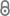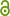# Exploiting structure in multiobjective optimization and optimal control

S. Peitz,   Exploiting Structure in Multiobjective Optimization and Optimal Control, 2017.Dissertation_Peitz.pdf 16.64 MBDissertation | Published | English
Department
Abstract
Multiobjective optimization plays an increasingly important role in modern applications, where several criteria are often of equal importance. The task in multiobjective optimization and multiobjective optimal control is therefore to compute the set of optimal compromises (the Pareto set) between the conflicting objectives. Since – in contrast to the solution of a single objective optimization problem – the Pareto set generally consists of an infinite number of solutions, the computational effort can quickly become challenging. This is even more the case when many problems have to be solved, when the number of objectives is high, or when the objectives are costly to evaluate. Consequently, this thesis is devoted to the identification and exploitation of structure both in the Pareto set and the dynamics of the underlying model as well as to the development of efficient algorithms for solving problems with additional parameters, with a high number of objectives or with PDE-constraints. These three challenges are addressed in three respective parts. In the first part, predictor-corrector methods are extended to entire Pareto sets. When certain smoothness assumptions are satisfied, then the set of parameter dependent Pareto sets possesses additional structure, i.e. it is a manifold. The tangent space can be approximated numerically which yields a direction for the predictor step. In the corrector step, the predicted set converges to the Pareto set at a new parameter value. The resulting algorithm is applied to an example from autonomous driving. In the second part, the hierarchical structure of Pareto sets is investigated. When considering a subset of the objectives, the resulting solution is a subset of the Pareto set of the original problem. Under additional smoothness assumptions, the respective subsets are located on the boundary of the Pareto set of the full problem. This way, the “skeleton” of a Pareto set can be computed and due to the exponential increase in computing time with the number of objectives, the computations of these subsets are significantly faster which is demonstrated using an example from industrial laundries. In the third part, PDE-constrained multiobjective optimal control problems are addressed by reduced order modeling methods. Reduced order models exploit the structure in the system dynamics, for example by describing the dynamics of only the most energetic modes. The model reduction introduces an error in both the function values and their gradients, which has to be taken into account in the development of algorithms. Both scalarization and set-oriented approaches are coupled with reduced order modeling. Convergence results are presented and the numerical benefit is investigated. The algorithms are applied to semi-linear heat flow problems as well as to the Navier-Stokes equations.
Publishing Year
LibreCat-ID

### Cite this

Peitz S.   Exploiting Structure in Multiobjective Optimization and Optimal Control.; 2017.
Peitz, S. (2017).   Exploiting structure in multiobjective optimization and optimal control.
@book{Peitz_2017, title={  Exploiting structure in multiobjective optimization and optimal control}, author={Peitz, Sebastian}, year={2017} }
Peitz, Sebastian.   Exploiting Structure in Multiobjective Optimization and Optimal Control, 2017.
S. Peitz,   Exploiting structure in multiobjective optimization and optimal control. 2017.
Peitz, Sebastian.   Exploiting Structure in Multiobjective Optimization and Optimal Control. 2017.
Main File(s)
File Name
Dissertation_Peitz.pdf 16.64 MB
Access LevelClosed Access
2020-03-13T12:52:50ZClosed Access